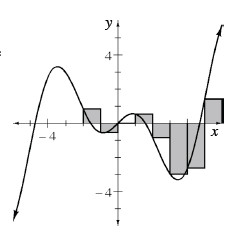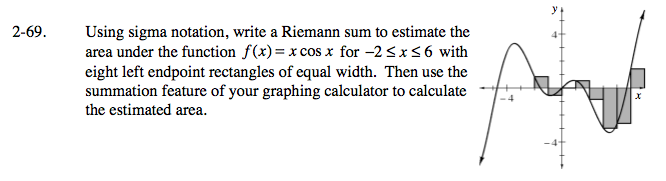### Home > CALC > Chapter 2 > Lesson 2.2.2 > Problem2-69

2-69.
1. Using sigma notation, write a Riemann sum to estimate the area under the function f(x) = x cos x for −2 ≤ x ≤ 6 with eight left endpoint rectangles of equal width. Then use the summation feature of your graphing calculator to calculate the estimated area. Homework Help ✎Since area of a rectangle = (base)(height), start by finding the base, or Δx of each rectangle. Divide the interval by the number of rectangles:

Next, find the height of each rectangle. The height is determined by f(axi), where a = starting value on the x-axis.
f((−2) + (1)i) = f(−2 + i)

Finally, use sigma notation to represent the sum of all eight rectangles. Since these are left-endpoint rectangles, the index will start at 0 and end at n − 1, where n = number of rectangles.

Use your calculator to evaluate this sum.